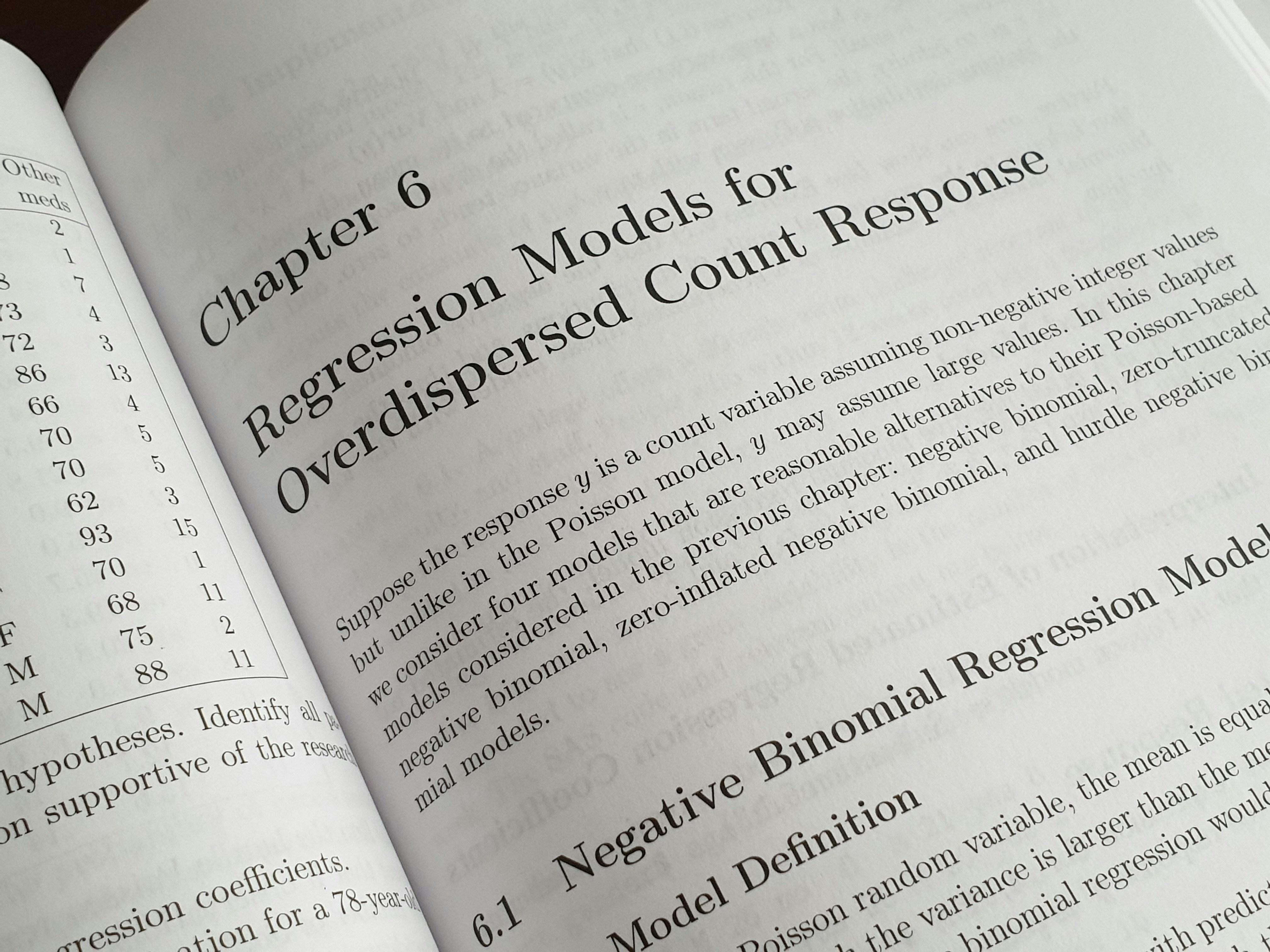多元线性回归

tags

date
Dec 9, 2018
source
status

多维特征（Multiple Features）

Size(feet2)
Number of bedrooms
Number of floors
Age of home(years)
price(\$1000)
5
1
45
460
3
2
40
232
3
2
30
315
2
1
36
178

💡

特征缩放（Feature Scaling）

min-max 归一化（Min-Max Normalization）：

💡
μ 为该特征所有样本数据的均值（mean）

Z-score 标准化（Z-score Standardization）：
💡
μ 为该特征所有样本数据的均值（mean）σ 为该特征所有样本数据的标准差（Standard Deviation）

# 单变量梯度下降
for ... in ... :
error = X.dot(theta) - y
theta -= (alpha / m) * np.sum(error)
theta -= (alpha / m) * np.sum(error.dot(X))
# theta 需要逐个运算
...

# 多变量梯度下降
for ... in ... :
theta -= (alpha / m) * (X.dot(theta) - y).dot(X)
# 直接将整个 theta 作为向量运算
...
💡

正规方程（Normal Equation）

• X 为训练集特征矩阵（包含了 ）。
• y 为训练集结果标签向量。
• Matlab 中的正规方程：pinv(X'*X)*X'*y
• Numpy 包中的正规方程：np.linalg.inv(X.T@X)@X.T@y
• TensorFlow 中的正规方程：
mat = tf.matmul(tf.transpose(X), X)
inv = tf.matrix_inverse(mat)
vec = tf.matmul(tf.transpose(X), y)
solution = tf.matmul(inv, vec)

《吴恩达机器学习系列课程》笔记